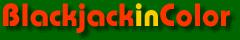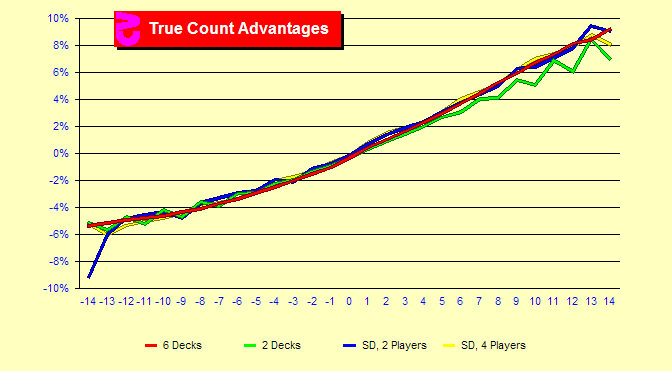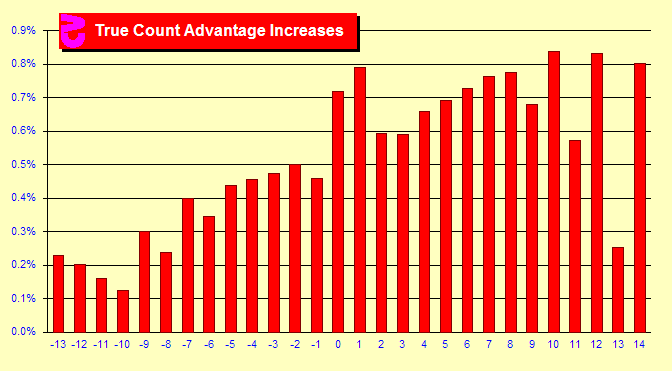###How valuable is each True Count?

Here we will look at the advantage at each count. That is the percentage gain you can expect on average from the initial Blackjack bet. Data for six decks, two decks and single-deck with two or four players are displayed. It can be seen that the number of decks does not have a large effect on the curve. If the rules were not adjusted by the casinos to provide about the same advantage for single-deck and shoes, the curves would separate, but would still have about the same shape. The upward trend appears fairly linear, but we will see that it is not. The angle of the curve is greater in the positive counts than in the negative counts. That is, the lines gently accelerate upwards. The advantages here are positive at counts of +1 and above and negative otherwise. But the +14 count is substantially more positive than the -14 count is negative.

###What is the change in advantage from TC to TC?

In this chart the increase in advantage at each count is displayed for six decks. For example, the bar at +1 shows that the advantage at a true count of +1 is .79% higher than the advantage at zero. A common card counting rule of thumb is that the increase at each count is about .5% for each count. As we can see this is a very rough estimate. This is not surprising since the effect of indexes kicks in at different points. Full indexes (over 100) were used in this chart. With a smaller number of indexes, the gains in advantage would be less. Few areas of Blackjack maintain simple linear relationships. Insurance is the most notable exception.

### Sim details

• Six decks, S17, DAS, LS, 4.81/6, Hi-Lo max indexes, trunc, half-deck
• Two decks, H17, DAS, 1.5/2, Hi-Lo Ill18 indexes, trunc, half-deck
• Single-deck, H17, 2 players, 4 rounds, Hi-Lo max indexes, trunc, quarter-deck
• Single-deck, H17, 4 players, 2 rounds, Hi-Lo max indexes, trunc, quarter-deck
• Ten billion rounds for six and two decks, five billion rounds for single-deck